Courses
Courses for Kids
Free study material
Free LIVE classes
More

## Easy Preparation for JEE Advanced with Class 12 Chemistry Chapter 14 Carbohydrates Important Questions

Last updated date: 27th Mar 2023
Total views: 84.9k
Views today: 0.62k

With regular practice, there is no doubt that anyone would be able to perform well in competitive examinations. For students who want to crack JEE Advanced, it is essential to prepare all the subjects carefully and Chemistry is one such subject. With the help of important questions and worksheets, students will get enough practice to score high marks in examinations. For example, the important questions on Carbohydrates have been created to introduce students to different sugars and fibres that are present inside the food items.

Carbohydrates are essential biomolecules that are present inside the body of any living being and help in the sustenance and growth of the organism. To understand the different types of carbohydrates and the characteristics that they have, practice from Carbohydrates important questions right now.

## Single Choice Type Question

1. The structure of D-(+)-glucose is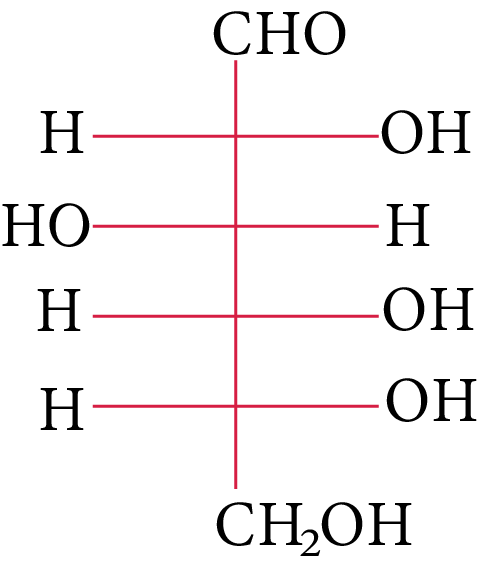D-(+)-glucose

The structure of L-(−)-glucose is       JEE Advanced 2015

(A)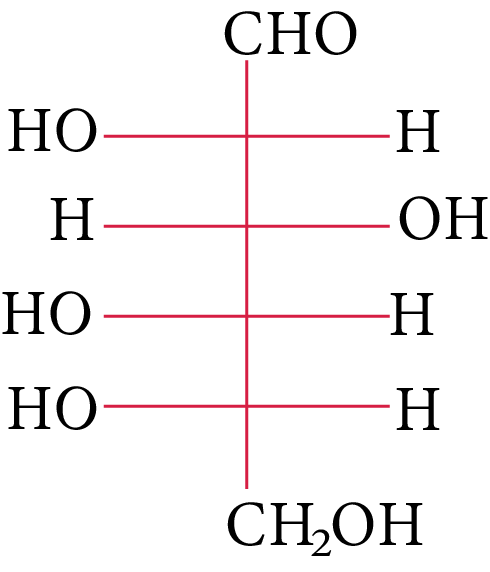L-(-)-glucose

(B)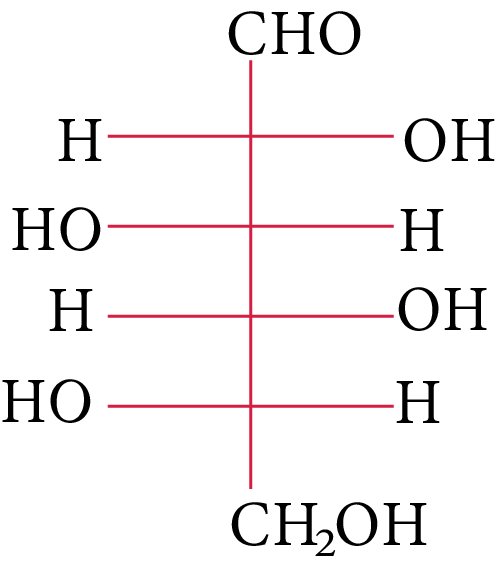D-(+)-maltose

(C)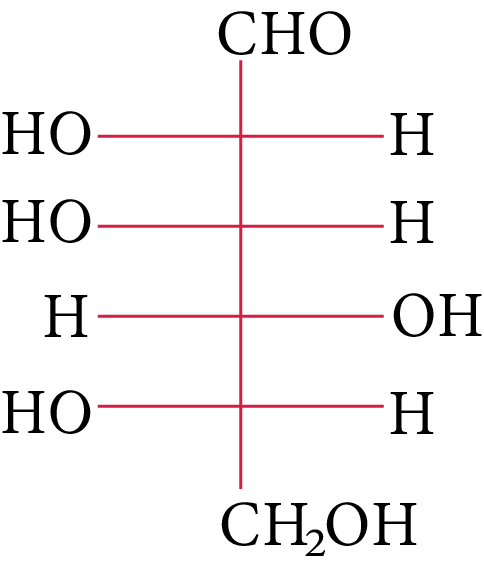D-(+)-lactose

(D)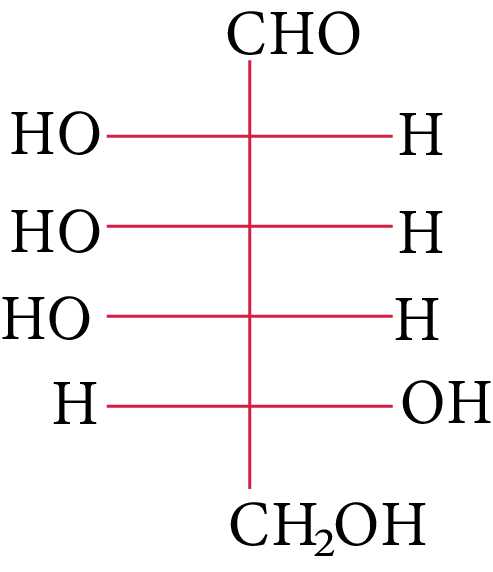D-(+)-galactose

1. The following carbohydrate is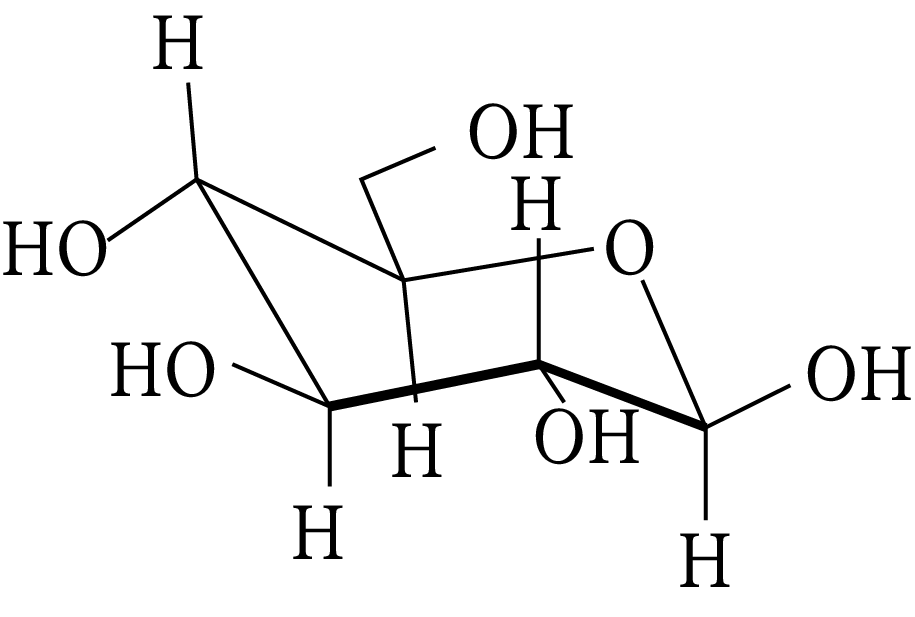Carbohydrate

JEE 2011

(A) a ketohexose

(B) an aldohexose

(C) an alpha furanose

(D) an alpha pyranose

1. The correct statement about the following disaccharide is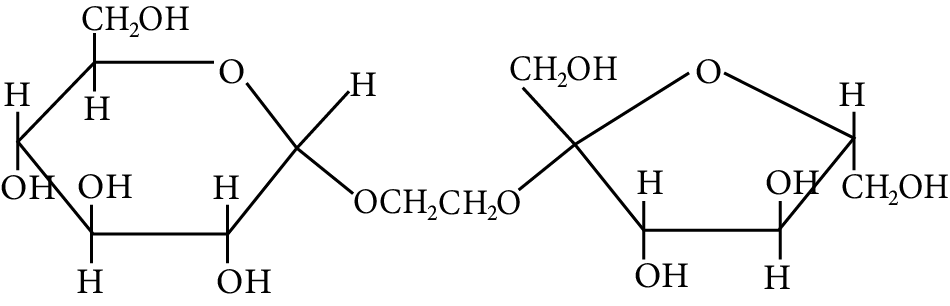Disaccharide

IIT 2010

(A) Ring (A) is pyranose with $\alpha$  glycosidic link

(B) Ring (A) is furanose with $\alpha$  glycosidic link

(C) Ring (B) is furanose with $\alpha$  glycosidic link

(D) Ring (B) is pyranose with $\beta$ -glycosidic link

1. The Fischer presentation of D-glucose is given below.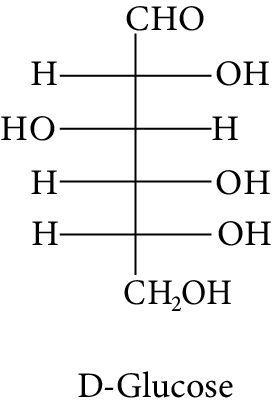D-Glucose

The correct structure (s) of beta glucopyranose is (are):

(A)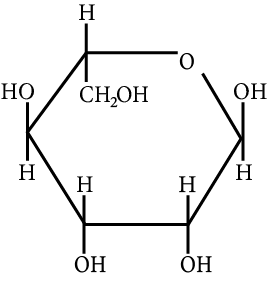Carbohydrate

(B)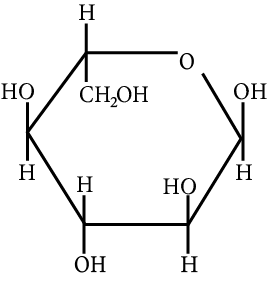Carbohydrate

(C)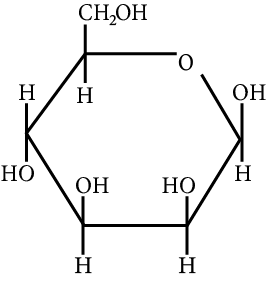Carbohydrate

(D)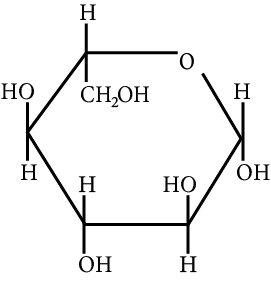Beta glucopyranose

1. The change in the optical rotation of freshly prepared solution of glucose is known as

AIEEE 2011

(A) tautomerism

(B) racemization

(C) specific rotation

(D) mutarotation

1. Accumulation of which of the following molecules in the muscles occurs as a result of vigorous exercise?

(A) Pyruvic acid

(B) L-lactic acid

(C) Glycogen

(D) Glucose

1. Which compounds will behave as reducing sugar in an aqueous KOH solution?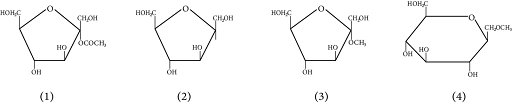Different Types of Carbohydrate

1. Complete hydrolysis of starch gives:

(A) glucose and fructose in equimolar amounts

(B) glucose only

(C) galactose and fructose in equimolar amounts

(D) glucose and galactose in equimolar amounts

1. Glycogen is a branched chain polymer of $\alpha - D - {\rm{glucose}}$  units in which chain is formed by ${{\rm{C}}_1}{\rm{ }} - {\rm{ }}{{\rm{C}}_4}$  glycosidic linkage whereas branching occurs by the formation of ${{\rm{C}}_1}{\rm{ }} - {\rm{ }}{{\rm{C}}_6}$  glycosidic linkage. Structure of glycogen is similar to __________.

(A) Amylose

(B) Amylopectin

(C) Cellulose

(D) Glucose

1.  Sucrose (cane sugar) is a disaccharide. One molecule of sucrose on hydrolysis gives _________.

(A) 2 molecules of glucose

(B) 2 molecules of glucose $$+$$ 1 molecule of fructose

(C) 1 molecule of glucose $$+$$ 1 molecule of fructose

(D) 2 molecules of fructose

1. Which of the following pairs represents anomers?

(A)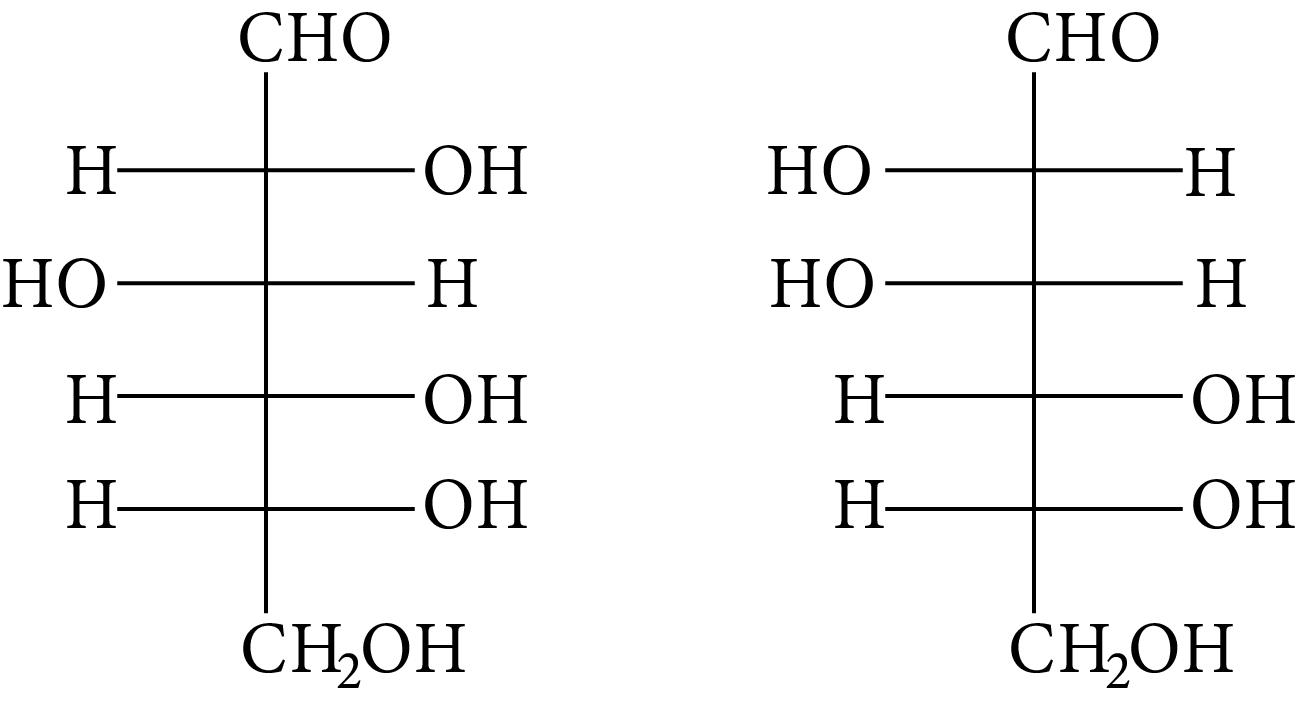(B)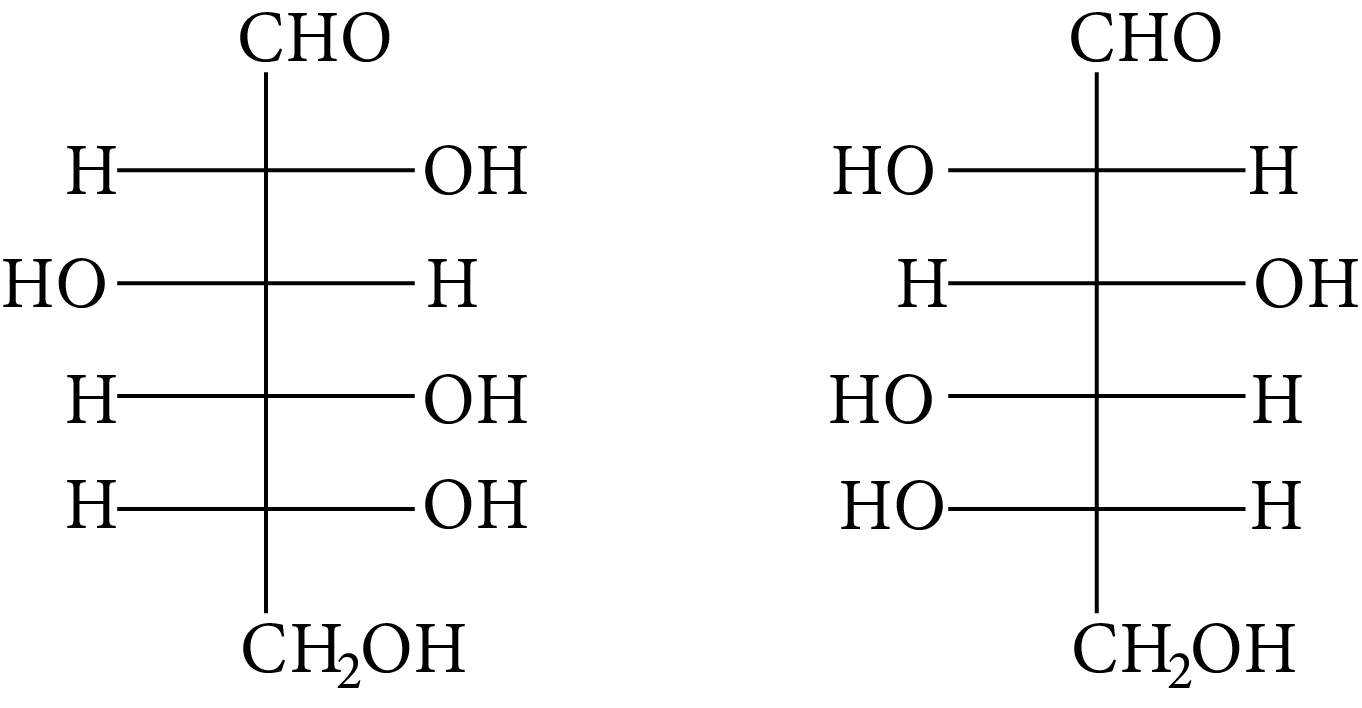(C)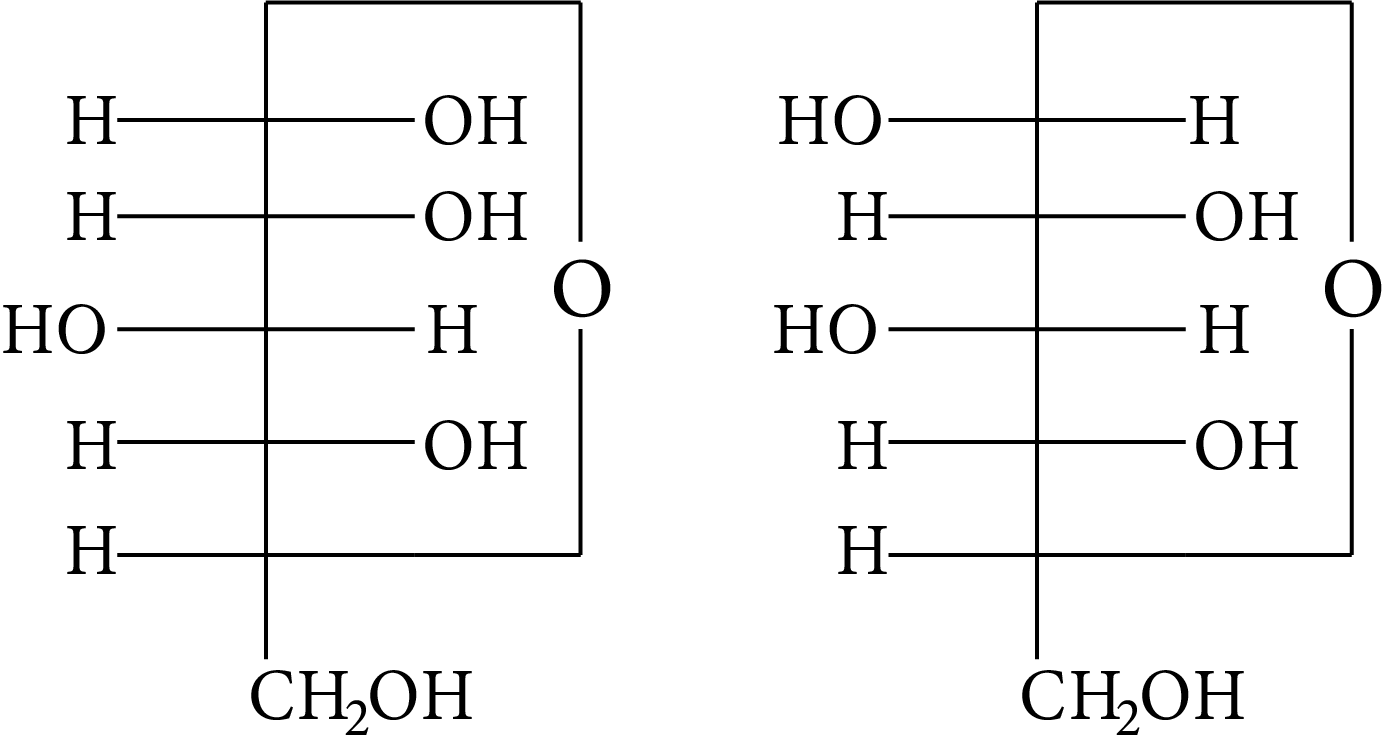(D)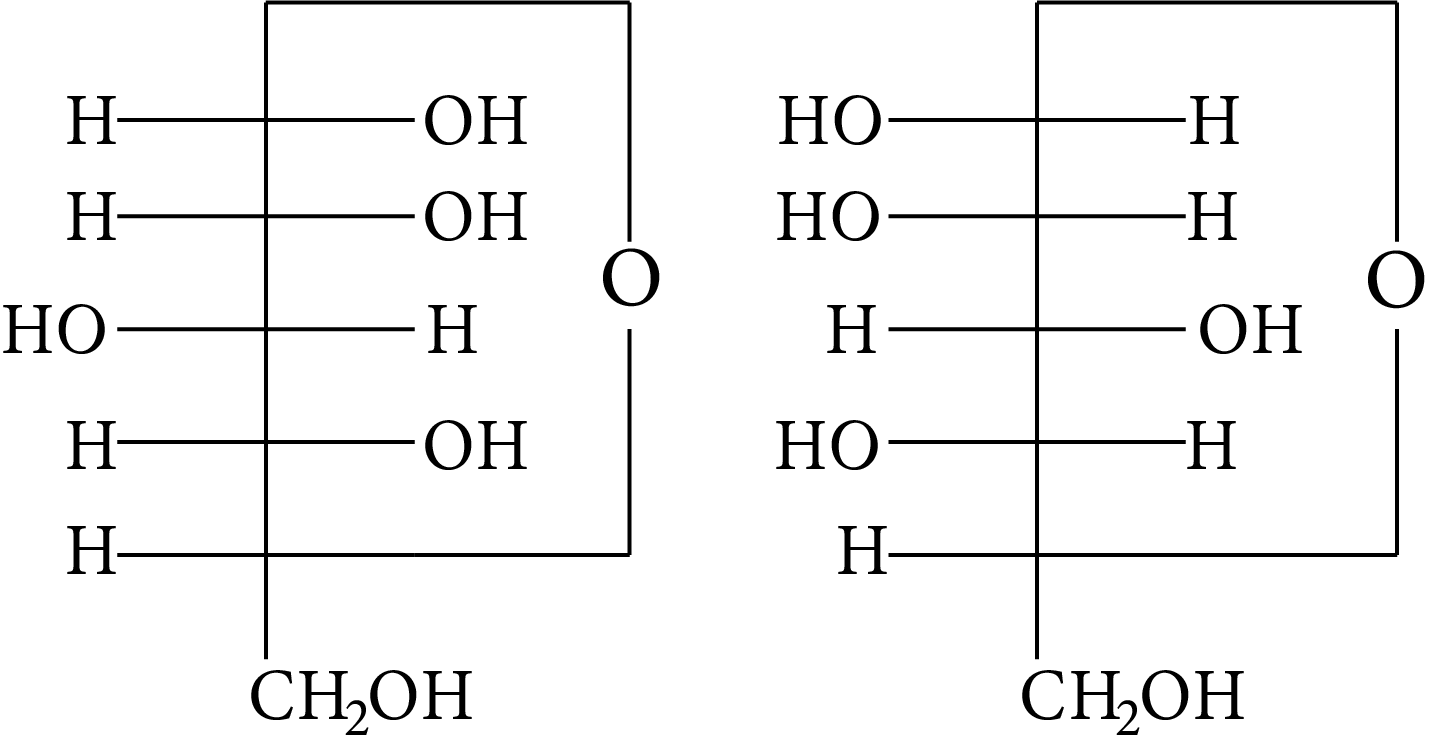1. In disaccharides, if the reducing groups of monosaccharides i.e. aldehydic or ketonic groups are bonded, these are non-reducing sugars. Which of the following disaccharide is a non-reducing sugar?

(A)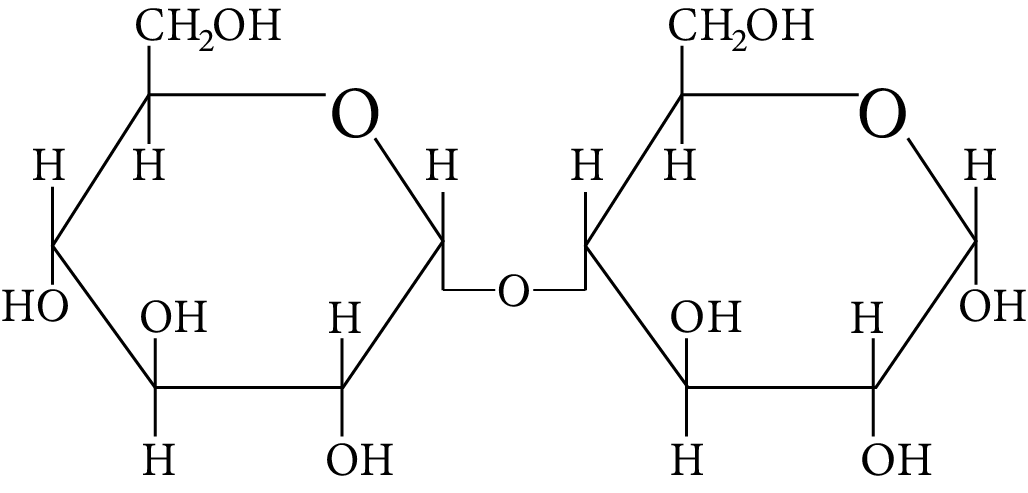Disaccharide

(B)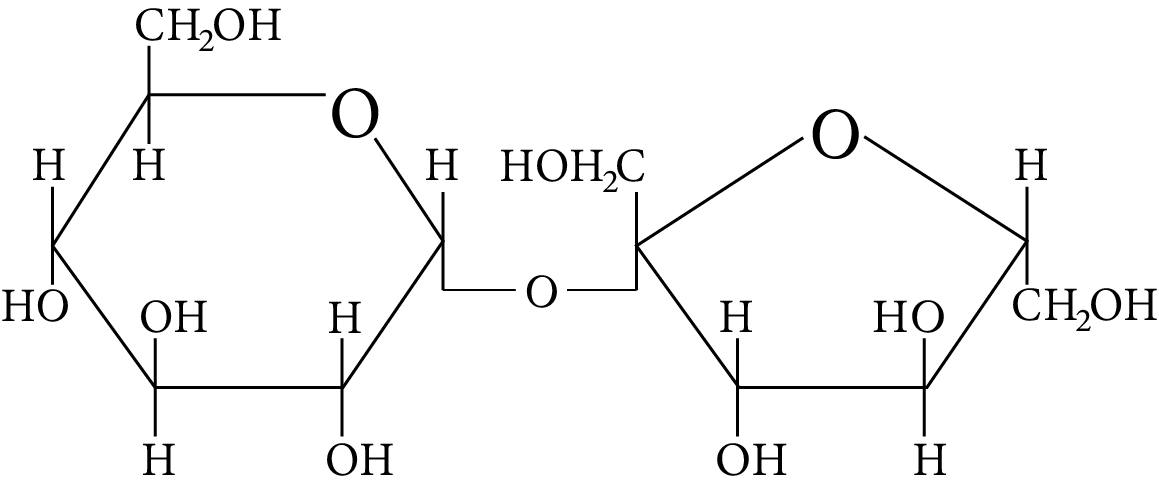Disaccharide

(C)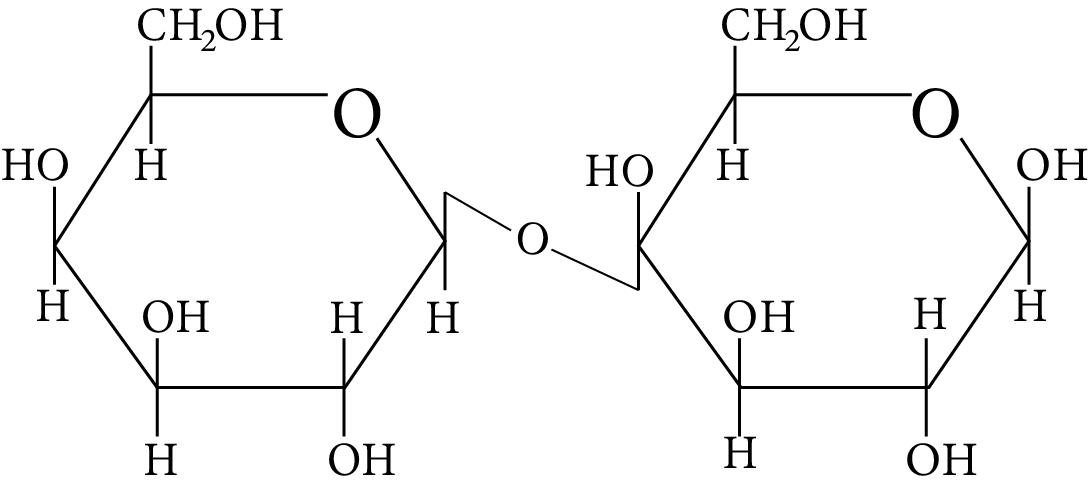Disaccharide

(D)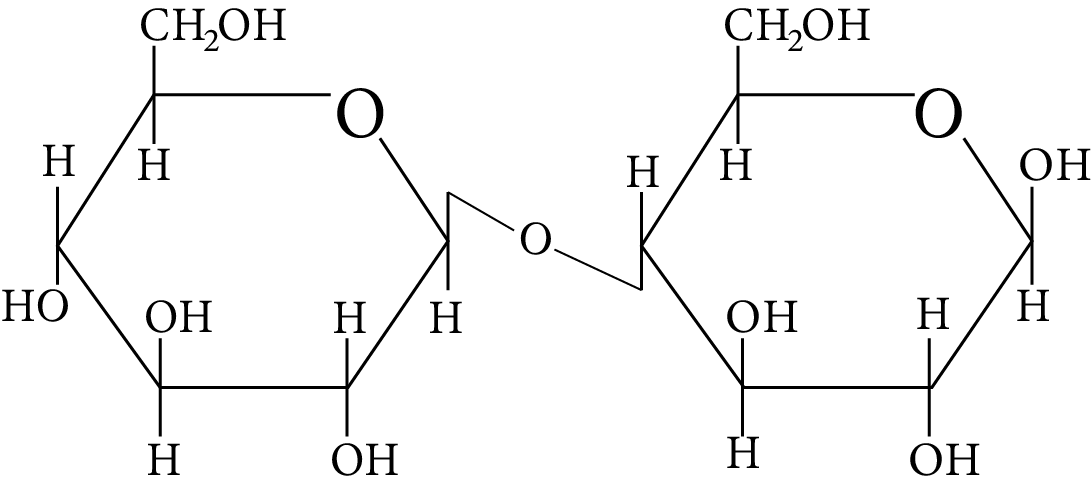Disaccharide

1.  Two nucleotides are joined by phosphodiester linkage to form a dinucleotide. Which pentose sugars and nucleotides' carbon atoms are connected by these linkages?

(A) $5\prime {\rm{ and }}3\prime$

(B) $1\prime {\rm{ and }}5\prime$

(C) $5\prime {\rm{ and }}5\prime$

(D) $3\prime {\rm{ and }}3\prime$

## Multiple Choice Type Question

1. Which of the following statements is not true about glucose?

(A) It is an aldohexose.

(B) On heating with ${\rm{HI}}$  it forms n-hexane.

(C) It is present in furanose form.

(D) It does not give $2,4 - {\rm{DNP}}$  test.

1. Carbohydrates are classified on the basis of their behaviour on hydrolysis and also as reducing or non- reducing sugar. Sucrose is a __________.

(A) monosaccharide

(B) disaccharide

(C) reducing sugar

(D) non-reducing sugar

1. Which of the following carbohydrates are branched polymers of glucose?

(A) Amylose

(B) Amylopectin

(C) Cellulose

(D) Glycogen

1. Which of the following statement(s) is(are) true?

(A) The two six-membered cyclic hemiacetal forms of D-(+)-glucose are called anomers

(B) Oxidation of glucose with bromine water gives glutamic acid

(C) Monosaccharides cannot be hydrolyzed to given polyhydroxy aldehydes and ketones

(D) Hydrolysis of sucrose gives dextrorotatory glucose and laevorotatory fructose

1. For "invert sugar", the correct statement(s) is(are)

(Given : specific rotations of (+)-sucrose, (+)-maltose, L-(−)-glucose and L-(+)-fructose in aqueous solution are $+66^{\circ},+140^{\circ},-52^{\circ} \text { and }+92^{\circ},$  respectively.)

(A) “Invert sugar” is prepared by acid catalysed hydrolysis of maltose.

(B) “Invert sugar” is an equimolar mixture of D-(+)-glucose and D-(+)-fructose.

(C) Specific rotation of “invert sugar” is -20.

(D) In reaction with Br2 water, “invert sugar” forms saccharic acid as one of the products.

1.  Given: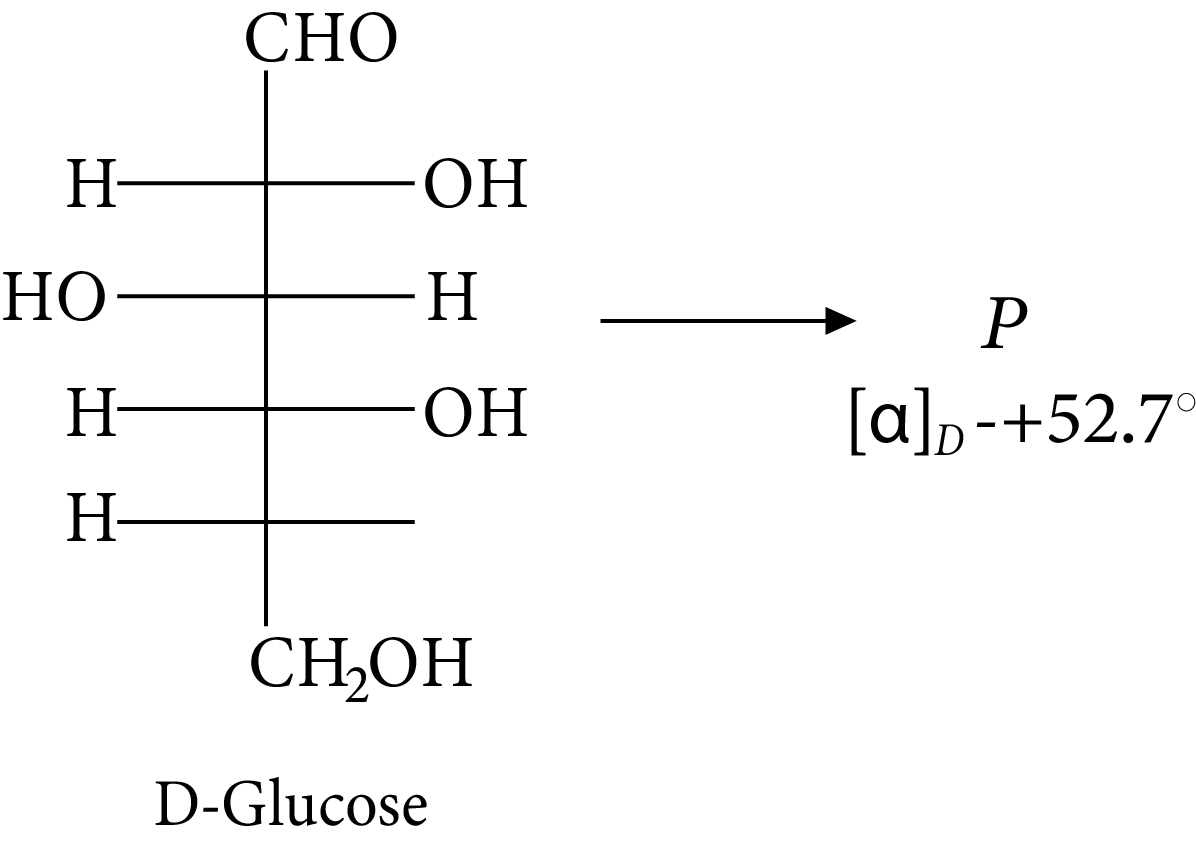D-glucose

The compound(s), which on reaction with HNO3 will give the product having degree of rotation,${[\alpha ]_D} = - {52.7^ \circ }$  is (are)

(A)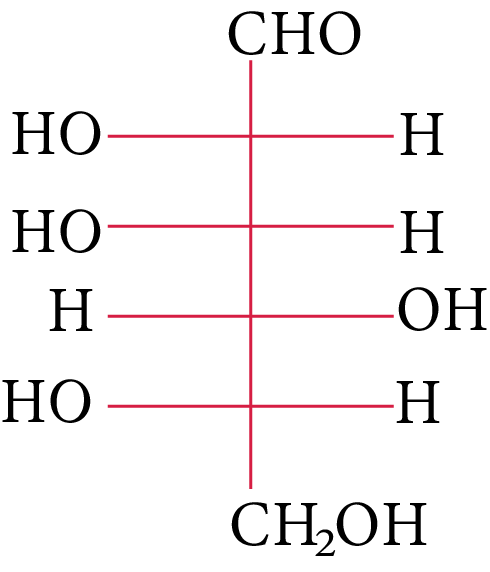(B)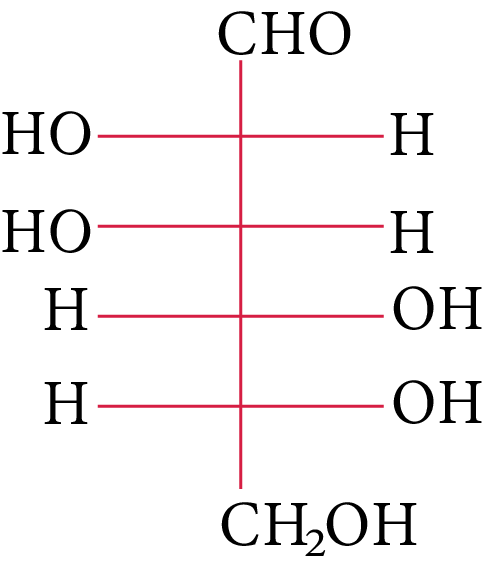(C)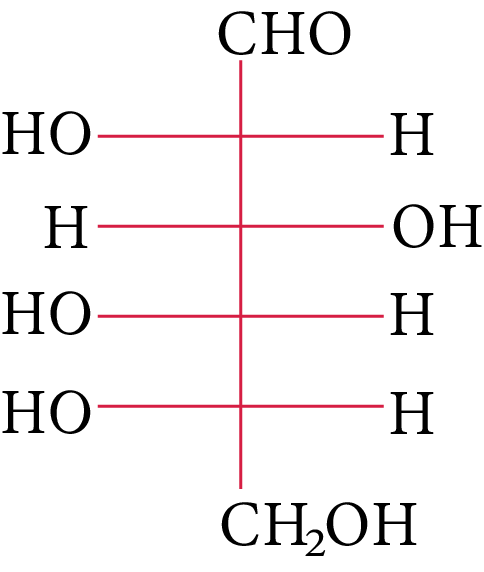(D) None of these

1. The two functional groups present in a typical carbohydrate are:

(A) $> {\rm{C}} = {\rm{O and - OH}}$

(B) ${\rm{ - OH and - CHO}}$

(C) ${\rm{ - OH and - COOH}}$

(D) ${\rm{ - CHO and - COOH}}$

1. Consider the following statements about glucose and state which of the following are correct.

(1) The glucose reaction with hydroxylamine and HCN confirms the presence of a carbonyl group in glucose.

(2) The reaction of glucose with nitric acid confirms the presence of a primary alcoholic group in glucose.

(3) The glucose reaction with HI suggests that all six carbon atoms are connected in a straight chain.

(A) (1)

(B) (2)

(C) (3)

(D) Only (1)

1.  Consider the following carbohydrates.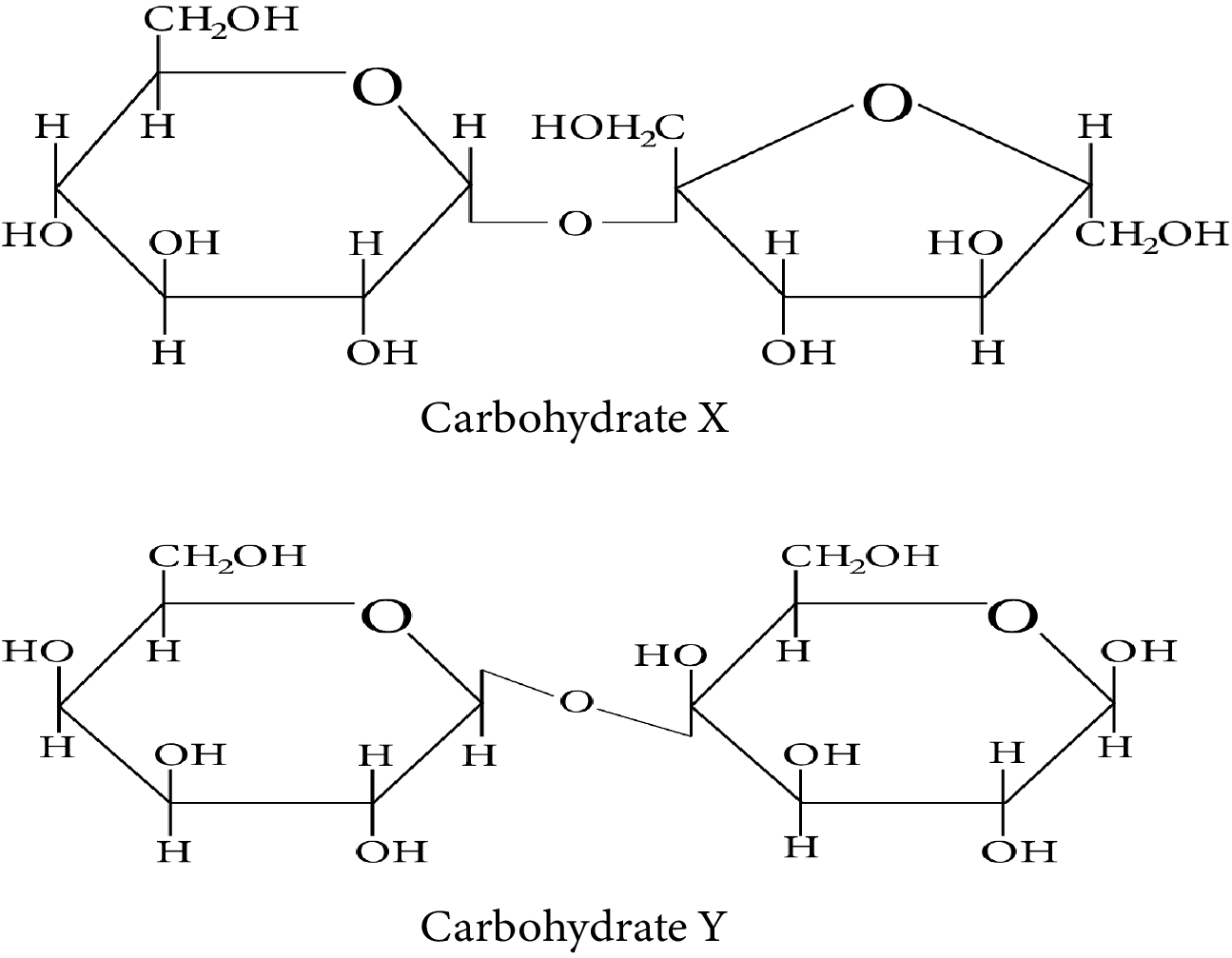Carbohydrate X and Y

Which of the following statement(s) is/are true regarding the two carbohydrates:

(i) X is a reducing sugar and Y is a non-reducing sugar.

(ii) X has ${\rm{\alpha }} - 1{\rm{, \beta }} - 2$  glycosidic linkage whereas Y has ${\rm{\beta }} - 1,4$  glycosidic linkage.

(iii) On treatment with sulfuric acid, X gives elemental carbon.

(iv) Y can have 4 anomeric forms.

(A) (i)

(B) (ii)

(C) (iii)

(D) (iv)

## Integer Type Questions

1. When the following aldohexose exists in its d-configuration, the total number of stereoisomers in its pyranose form is

1.  The number of chiral carbons present in sucrose is _____.

JEE Main - 2020

1. Synthesis of each molecule of glucose in photosynthesis involves:

## Solutions

1. (A)

The OH group linked to the final stereocenter is on the right side of D-(+)-glucose while it is on the left side of L-(-)-glucose. The L-(-)-glucose molecule isL-(-)-glucose

1. (B)

The carbohydrate is hexose because it has six carbons. Additionally, the first carbon, anomeric carbon, contains hydrogen. The new chiral centre created by cyclization is called anomeric C (first carbon).

It is the cyclic form of D-glucose, and as glucose functions as an aldehyde, it is also known as aldohexose.

1. (A)

Pyranose is the name given to the six-membered cyclic ether, whereas furanose is the name given to the five-membered cyclic ether.

As a result, ring (a) is a pyranose and possesses an ether linkage, also known as $\alpha$ -glycosidic bond in carbohydrate chemistry, at the $\alpha$ -position.

1. (D)

The energy source D-glucopyranose, a synthetic simple monosaccharide, has a beta isoform called beta-D-glucopyranose.

1. (D)

The freshly generated glucose solution equilibrium results in an uneven combination of (+) and (-) glucose. This occurrence is known as mutarotation.

1. (B)

Exercise that is intense causes L-lactic acid to build up in the muscles.

Anaerobic respiration, or the breakdown of carbohydrates in the absence of oxygen, is to blame.

1. (A)

The following reaction occurs when ester is present in an aqueous KOH solution and undergoes the SNAE reaction.

A hydroxy carbonyl compound is created in the aforementioned chemical when aq. KOH (SNAE) reaction occurs, which results in a positive Tollens test result. As a result, this substance behaves like a reducing sugar in an aqueous KOH solution.

1. (B)

The monomer of starch, which is glucose, is a combination of the polysaccharides amylose and amylopectin. So, upon total hydrolysis, it solely yields glucose.

1. (B)

Glycogen is similar to the structure of amylopectin. It is an $\alpha - D - {\rm{glucose}}$  unit branched chain polymer which is created by the glycosidic linkage of ${{\rm{C}}_1}{\rm{ }} - {\rm{ }}{{\rm{C}}_4}$  and branching by ${{\rm{C}}_1}{\rm{ }} - {\rm{ }}{{\rm{C}}_6}$  glycosidic linkage.

Thus, option (B) is correct.

1. (C)

Sucrose (also known as cane sugar) is a type of disaccharide. When sucrose is hydrolyzed, one molecule of glucose and one molecule of fructose are produced.

1. (C)

At ${\rm{C}} - 1,$  anomers have a distinct configuration. If hydroxyl group is present on the right side of anomeric carbon, it is referred to as the $\alpha -$ form whereas if hydroxyl group is present on the left side, it is referred to as the $\beta -$ form. Therefore, among all the given pairs, option (C) represents anomers.

1. (B)

Among all the given disaccharides, the structure given in option (B) represents sucrose, in which $\alpha - D -$  glucose and $\beta - D -$ fructose are linked by a ${{\rm{C}}_1} - {{\rm{C}}_2}$  glycosidic bond.

Due to the involvement of the reducing groups of glucose and fructose in the formation of glycosidic bonds, this is classified as a non-reducing sugar.Sucrose

Thus, option (B) is correct.

1. (A)

The two nucleotides are linked together by a phosphodiester to form a dinucleotide. The pentose sugars of nucleotides have 5′ and 3′ linkages. Thus, option (A) is correct.

1. (A, and B)

Glucose exists as pyranose, which has a five-membered ring structure. As a result, the statement in option (C) is incorrect.

Glucose is a monosaccharide containing six carbon atoms and an aldehyde group. As a result, it's a type of aldohexose. As a result, the statement in option (A) is correct.

When glucose is heated with, n-hexane is produced. Due to this process, all six carbon atoms in glucose are bonded together in a straight chain. As a result, the statement in option (B) is correct.

The aldehyde group is not free in the cyclic structure. As a result, it does not offer a 2-4 DNP test. Thus, the statement given in option (D) is correct.

1. (B and D)

Sucrose is a common disaccharide which on hydrolysis produces an equimolar combination of $D - \left( + \right) -$ glucose and $D - \left( - \right) -$ fructose. A glycosidic link between C1 of $\alpha -$ glucose and C2 of $\beta -$ fructose holds these two monosaccharides together. Because the reducing groups of glucose and fructose are involved in forming glycosidic bonds, sucrose is a non-reducing sugar. Thus, options (B) and (D) are correct.

1. (B and D)

Amylopectin and Glycogen are the correct choices because they are types of carbohydrate storage in animal tissues, whereas amylose is made up of $80 - 85{\rm{ \% }}$  starch. It is also a branched polymer of glucose that is linked together via ${\rm{C1}} - {\rm{C6}}$ glycosidic linkage.

Thus, options (B) and (D) are correct.

1. (A, C and D)

(A) Anomers are two six-membered cyclic hemiacetal forms of D-(+)-glucose. Both are anomers.

(B) Gluconic acid is produced when glucose is oxidised in the presence of ${\rm{B}}{{\rm{r}}_{\rm{2}}}$  water.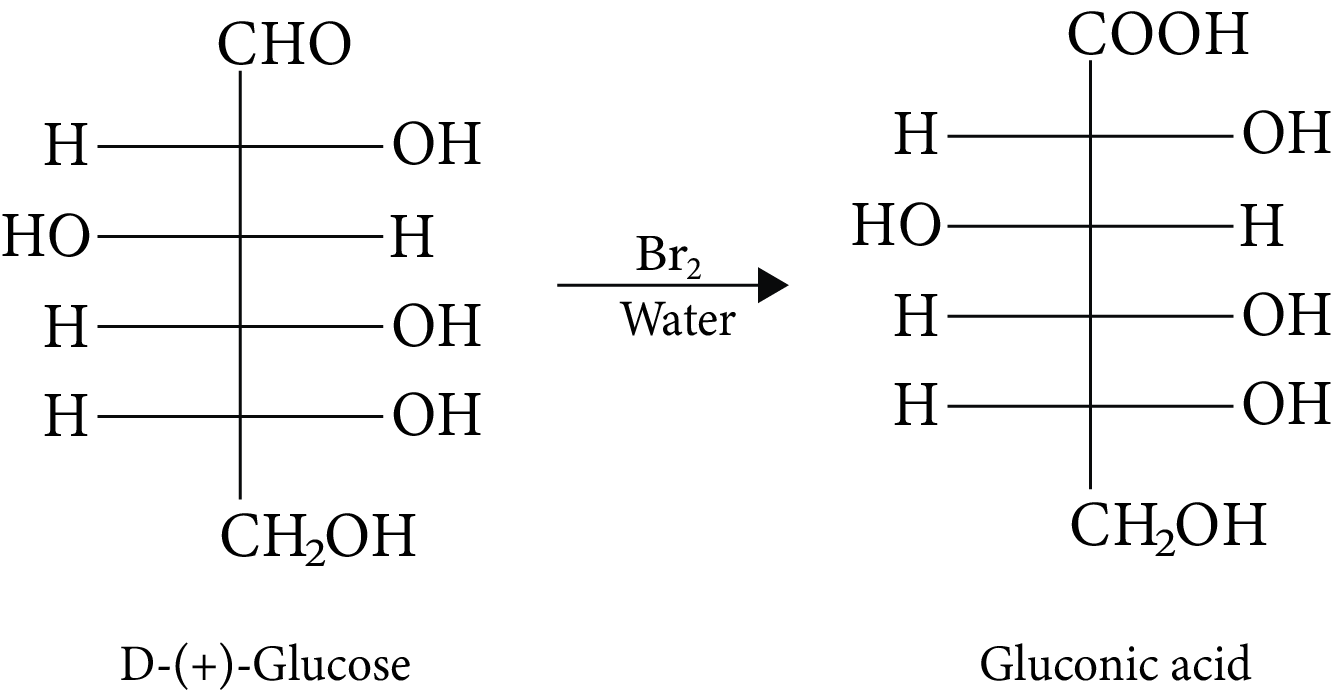D-(+)-Glucose and Gluconic Acid

(C) It is impossible to hydrolyze monosaccharides into polyhydroxy aldehydes and ketones.

(D) D-glucose and L-fructose are produced during sucrose hydrolysis.

1. (B and C)

The hydrolysis of sucrose under acid catalysis produces invert sugar.

The specific rotation of invert sugar is $\begin{array}{c}{\left[ \alpha \right]_{{\rm{mix}}}} = 0.5 \times \left( { + 52} \right) + 0.5 \times \left( { - 92} \right)\\ = + {\rm{ }}26 - 46{\rm{ }}\\ = - {20^ \circ }\end{array}$

When invert sugar reacts with ${\rm{B}}{{\rm{r}}_{\rm{2}}}$  water, one of the results is gluconic acid. Fructose is not oxidised by ${\rm{B}}{{\rm{r}}_{\rm{2}}}$  water, which converts glucose into gluconic acid.

1. (c)

In its reaction with ${\rm{HN}}{{\rm{O}}_{\rm{3}}}{\rm{,}}$  monosaccharide oxidised to produce carboxylic acids. Along with aldehyde, primary alcohols also oxidise to carboxylic acid.

Rotation of the product (P) enantiomer is ${\rm{52}}{\rm{.}}{{\rm{7}}^{\rm{0}}}{\rm{.}}$

1. (A and B)

Carbohydrates are polyhydroxy aldehydes, polyhydroxy ketones, or substances that, upon hydrolysis, provide both aldehyde and ketonic groups. As a result, (carbonyl) aldehyde, ketone, and hydroxyl groups are the main functional groups.

1. (A, B and C)

Glucose is one of the important carbohydrates with the molecular formula of ${{\rm{C}}_{\rm{6}}}{{\rm{H}}_{{\rm{12}}}}{{\rm{O}}_{\rm{6}}}.$  It is also referred as aldohexose because it contains six carbon atoms and an aldehyde group.

When glucose is treated with hydroxylamine, an oxime is formed. When glucose is treated with hydrogen cyanide, cyanohydrin is formed.

These two statements suggest that glucose contains a carbonyl group.

Thus, statement (1) is correct.

Glucose gets oxidised in the presence of nitric acid and results in the formation of saccharic acid.

Here, the number of carbon atoms remains the same, which shows the presence of the primary alcoholic group in glucose.

Thus, statement (2) is also correct.

Glucose on prolonged heating with HI and red phosphorus yields n-hexane.

This statement suggests that all six carbon atoms are linked in a straight chain.

Thus, statement (3) is also correct.

1. (B and C)

Compound X contains two subunits, glucose and fructose. Therefore, X is sucrose which is one of the common disaccharides. On hydrolysis, it gives equimolar mixture of $D - \left( - \right){\rm{fructose}}$  and $D - \left( + \right){\rm{glucose}}{\rm{.}}$

The two monosaccharides, glucose and fructose, are joined by glycosidic linkage between C-1 of ${\rm{\alpha }} - D - {\rm{glucose}}$  and C-2 of ${\rm{\beta }} - D - {\rm{fructose}}{\rm{.}}$

Since the reducing groups of fructose and glucose are involved in the formation of glycosidic bonds, the sugar is non-reducing in nature. Therefore, carbohydrate X is a non-reducing sugar.

Compound Y contains two subunits, galactose and glucose. Therefore, Y is Lactose.

Lactose is a disaccharide which is found in milk. It is also known as milk sugar. It consists of glucose and galactose subunits, joined by glycosidic linkage between C-1 of ${\rm{\beta }} - D - {\rm{galactose}}$  and C-4 of ${\rm{\beta }} - D - {\rm{glucose}}{\rm{.}}$

A free aldehyde group may be formed in Lactose at C-1 of the glucose unit. Hence, this sugar is reduced in nature. Therefore, carbohydrate Y is a reducing sugar.

The systematic name of Lactose is ${\rm{\beta }} - D - {\rm{galactopyranosyl}} - \left( {1 \to 4} \right) - D - {\rm{glucose}}{\rm{.}}$  The glucose can have ${\rm{\alpha }} - {\rm{pyronose}}$  and ${\rm{\beta }} - {\rm{pyronose}}$  forms whereas the galactose can only have ${\rm{\beta }} - {\rm{pyronose}}$  form. Hence, ${\rm{\alpha }} - {\rm{Lactose}}$  and ${\rm{\beta }} - {\rm{Lactose}}$  are two possible anomeric forms of glucopyranose ring alone.

1. 8

The three chiral carbon configurations (starting) can be altered while retaining the d-configuration. Consequently, the total number of d-pyranose stereoisomers.

So, 8 is the correct answer.

1. 9

A carbon atom that is joined to four different kinds of atoms or groups of atoms is said to be chiral. So, the total number of chiral carbons present in sucrose is 9.

1. 18

$\begin{array}{*{20}{l}}{{\rm{12}}{{\rm{H}}_{\rm{2}}}{\rm{O + 12 NADP + 18 ADP}} \to {\rm{6}}{{\rm{O}}_{\rm{2}}}{\rm{ + 18 ATP + 12 NADPH }}\left( {{\rm{ light \ reaction}}} \right)} \\ \\ {{\rm{6C}}{{\rm{O}}_{\rm{2}}}{\rm{ + 12 NADPH + 18 ATP}} \to {{\rm{C}}_{\rm{6}}}{{\rm{H}}_{{\rm{12}}}}{{\rm{O}}_{\rm{6}}}{\rm{ + 12 NADP + 18 ADP + 6}}{{\rm{H}}_{\rm{2}}}{\rm{O }}\left( {{\rm{dark \ reaction}}} \right)} \\ \\ {{\rm{6C}}{{\rm{O}}_{\rm{2}}}{\rm{ + 6}}{{\rm{H}}_{\rm{2}}}{\rm{O}} \to {{\rm{C}}_{\rm{6}}}{{\rm{H}}_{{\rm{12}}}}{{\rm{O}}_{\rm{6}}}{\rm{ + 6}}{{\rm{O}}_{\rm{2}}}{\rm{}}\left( {{\rm{Net \ reaction}}} \right)}\end{array}$

So, 18 molecules of ATP are involved in synthesising each molecule of glucose in photosynthesis.

## Importance of JEE Advanced Chemistry Carbohydrates Important Questions

The topic of carbohydrates has been introduced in the 14th chapter of the Class 12 chemistry syllabus. This topic is a part of the Biomolecules chapter that discusses different enzymes, lipids, proteins, and other elements that are responsible for the growth of living organisms. By reading the chapter, students can understand what carbohydrates are and what types exist in nature.

They will learn important terms such as monosaccharides, polysaccharides, oligosaccharides, etc. Students can further educate themselves on the different types of monosaccharides and reducing sugars. They will learn about the preparation of glucose from starch as well as sucrose.

The chapter also discusses different reactions in the formation of carbohydrates such as oxidation, reduction, acetylation, oxime formation, cyanohydrins formation, formation of Osazone, and so much more. They can practice these reactions and solve questions related to Carbohydrates Class 12 Chemistry.

Students will also learn about the cyclic structure of glucose and how it differs from fructose in molecular formula, melting point, optical nature, and other parameters. They will also learn about different disaccharides and polysaccharides that exist in nature. Using Carbohydrates JEE Advanced questions for practice, students will understand the reactions and solve different questions from the chapter. These questions also help in developing the theoretical as well as practical knowledge of students. Hence, having a strong conceptual foundation becomes easier with these questions.

## Benefits of Vedantu’s Important Questions for Carbohydrates

• The subject experts at Vedantu have included important questions related to the topic of carbohydrates in the chapter for the understanding of children. Every single question that is included in the PDF files is the one that might be asked in the upcoming JEE Advanced exam. So, students can prepare for the JEE Advanced exams easily with these questions.

• By solving JEE Advanced Chemistry carbohydrates important questions, students can familiarise themselves with different topics from the chapter, thus establishing a good grasp of the chapter. The experts at Vedantu have explained every single problem in detail and that too in a simple language for students to understand.

• By studying the question paper prepared by experts at Vedantu, students are most likely to understand the examination pattern of JEE Advanced. They will have an idea about the questions asked in the exams as well as the marking scheme associated with them.

Now you can download the JEE Advanced carbohydrates important questions from Vedantu and start your preparation for competitive exams early on. These PDF files are free to download and including these into your study routine will make the preparation even faster and easier.

1. What are the different types of carbohydrates?

The different types of carbohydrates are monosaccharides, polysaccharides, and oligosaccharides.

2. What are reducing sugars?

Reducing sugars are compounds that include a ketonic or aldehydic group in the hemiketal or hemiacetal forms. These compounds can reduce Fehlmg’s Solution or Tokken’s reagent.

3. What is the polysaccharide that can be found in the livers of different animals?

The reserve polysaccharide in an animal’s liver is called Glycogen. This polysaccharide is created with Hepatic glycogen. It is also produced in the muscles of different organisms.

4. Why doesn’t Glucose react with Sodium hydrogen sulphite even though it is an aldose sugar?

There is an aldehyde group that is present in the structure of glucose. The glucose isn’t about to react with the sodium hydrogen sulphite and hence isn’t able to produce bisulphate addition products. This kind of reaction can only take place when a free aldehyde group. But there isn’t any free –CHO group in the structure of glucose. Hence, the reaction doesn’t take place.